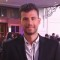Home » Core Java » util » Arrays » Sort arrays exampleIlias is a software developer turned online entrepreneur. He is co-founder and Executive Editor at Java Code Geeks.

# Sort arrays example

This is an example of how to sort arrays. We are using an `int` array in the example, but the same API applies to any type of arrays e.g. `byte[]`, `char[]`, `double[]`, `float[]`, `long[]`, `short[]`. Sorting an int array implies that you should:

• Create an `int` array with elements.
• Invoke `sort(int[] a)` API method of Arrays . It sorts an array in ascending order based on quicksort algorithm.
• We can fully sort an array by using `sort(array)` method of Arrays or we can partially sort an array by using `sort(array, startIndex, endIndex)` API method of Arrays where `startIndex` is inclusive and `endIndex` is exclusive. We can print the array’s elements before and after sorting in order to check the elements’ sorting.

Let’s take a look at the code snippet that follows:

```package com.javacodegeeks.snippets.core;

import java.util.Arrays;

public class SortArrayExample {

public static void main(String[] args) {

/*

Please note that the same API applies to any type of arrays e.g.

byte[], char[], double[], float[], long[], short[]
*/

// Create int array
int intArray[] = {1,4,3,5,2};

System.out.print("Array prior sorting :");
for(int i=0; i < intArray.length ; i++)

System.out.print("  "  + intArray[i]);

/*
Arrays.sort() method sorts an array in ascending order based on quicksort
algorithm. We can fully sort an array by using Arrays.sort(array) operation
or we can partially sort an array by using Arrays.sort(array, startIndex, endIndex)
operation where startIndex is inclusive and endIndex is exclusive
*/
Arrays.sort(intArray);

System.out.print("nArray after full sort :");
for(int i=0; i < intArray.length ; i++)

System.out.print("  "  + intArray[i]);

}
}
```

Output:

``````Array prior sorting :  1  4  3  5  2
Array after full sort :  1  2  3  4  5```
```

This was an example of how to sort an array in Java.

# Do you want to know how to develop your skillset to become a Java Rockstar?

## Subscribe to our newsletter to start Rocking right now!

### and many more ....